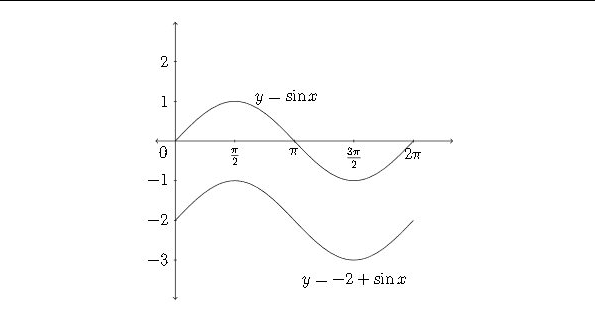# 9.3: The Vertical Shift of a Trigonometric Function

$$\newcommand{\vecs}{\overset { \rightharpoonup} {\mathbf{#1}} }$$ $$\newcommand{\vecd}{\overset{-\!-\!\rightharpoonup}{\vphantom{a}\smash {#1}}}$$$$\newcommand{\id}{\mathrm{id}}$$ $$\newcommand{\Span}{\mathrm{span}}$$ $$\newcommand{\kernel}{\mathrm{null}\,}$$ $$\newcommand{\range}{\mathrm{range}\,}$$ $$\newcommand{\RealPart}{\mathrm{Re}}$$ $$\newcommand{\ImaginaryPart}{\mathrm{Im}}$$ $$\newcommand{\Argument}{\mathrm{Arg}}$$ $$\newcommand{\norm}{\| #1 \|}$$ $$\newcommand{\inner}{\langle #1, #2 \rangle}$$ $$\newcommand{\Span}{\mathrm{span}}$$ $$\newcommand{\id}{\mathrm{id}}$$ $$\newcommand{\Span}{\mathrm{span}}$$ $$\newcommand{\kernel}{\mathrm{null}\,}$$ $$\newcommand{\range}{\mathrm{range}\,}$$ $$\newcommand{\RealPart}{\mathrm{Re}}$$ $$\newcommand{\ImaginaryPart}{\mathrm{Im}}$$ $$\newcommand{\Argument}{\mathrm{Arg}}$$ $$\newcommand{\norm}{\| #1 \|}$$ $$\newcommand{\inner}{\langle #1, #2 \rangle}$$ $$\newcommand{\Span}{\mathrm{span}}$$$$\newcommand{\AA}{\unicode[.8,0]{x212B}}$$

If a constant is added or subtracted to a trigonometric function, this will affect the $$y$$ -values of the function If we consider the function $$y-5+\sin x,$$ then each of the standard $$y$$ -values would have 5 added to if, which would shift the graph up 5 units.
The chart below considers just the quadrantal values for the sine function:
\begin{tabular}{|c|c|c|}
\hline$$\theta$$ & $$\sin \theta$$ & $$5+\sin \theta$$ \\
\hline 0 & 0 & 5 \\
\hline$$\pi / 2$$ & 1 & 6 \\
\hline$$\pi$$ & 0 & 5 \\
\hline $$3 \pi / 2$$ & -1 & 4 \\
\hline $$2 \pi$$ & 0 & 5 \\
\hline
\end{tabular}Sometimes the $$x$$ -axis is drawn through the line that is the new "zero" or "midline" for the function - in this case it would be $$y-5$$Likewrise, a negative constant would move the graph down, as each y-value would be less than the corresponding $$y$$ -value in the standard sine functionIn the previous examples the constant has been written in front of the sine function for clarity. Often the constant is written after the function:
$\begin{array}{c} y-\sin x+5 \\ \mathrm{or} \\ y-\sin x-2 \end{array}$

We have now examined three of the four transformations of trigonometric functions that are discussed in this chapter - amplitude, period and vertical shift. A general equation for a sinusoid that involves these three transformations would be:
$y=A \sin (B x)+D$ Or
$y=A \cos (B x)+D$ In determining an equation from a graph that involves a vertical shift, the value of $$A$$ will be half the distance between the maximum and minimum values:
$A=\frac{m a x-m i n}{2}$ and the value of $$D$$ will be the average of the maximum and minimum values:
$D=\frac{m a x+m i n}{2}$

Example
Determine an equation that satisfies the given graph.In this graph, the maximum $$y$$ -value is 6 and the minimum $$y$$ -value is $$-2 .$$ The average of these two:
$\frac{m a x+m i n}{2}=\frac{6+(-2)}{2}=\frac{4}{2}=2=D$ is the value of $$D,$$ the vertical shift.
The distance between 6 and -2 is $$6-(-2)=8 .$$ Half the distance between the $$\max$$ and $$\min$$ is $$4,$$ which is the value of $$A$$
$\frac{m a x-m i n}{2}=\frac{6-(-2)}{2}=\frac{8}{2}=4=A$

The graph completes one full cycle between 0 and $$3 \pi,$$ so the period would be $$3 \pi$$ and the value of $$B$$ would be $$B=\frac{2 \pi}{P}=\frac{2 \pi}{3 \pi}=\frac{2}{3}=B .$$ So a correct equation for the graph would be:
$y=4 \sin \frac{2}{3} x+2$

Exercises 2.3
Determine the Amplitude, Period and Vertical Shift for each function below and graph one period of the function. Identify the important points on the $$x$$ and $$y$$ axes.
1. $$\quad y=\sin x+1$$
2. $$\quad y=\cos x-1$$
3. $$\quad y=2 \cos x-\frac{1}{2}$$
4. $$\quad y=5 \sin x+4$$
5. $$\quad y=-\sin \left(\frac{1}{4} x\right)+1$$
6. $$\quad y=-\cos (2 x)+7$$
7. $$\quad y=\frac{1}{3} \sin (\pi x)-4$$
8. $$\quad y=-\frac{1}{2} \cos (2 \pi x)+2$$
9. $$\quad y=5 \cos \left(\frac{1}{2} x\right)+1$$
$$10 . \quad y=4 \sin \left(\frac{1}{3} x\right)-1$$
11. $$\quad y=3 \cos x+2$$
12. $$\quad y=2 \sin x+3$$
13. $$\quad y=2-4 \cos (3 x)$$
14. $$\quad y=5-3 \sin (2 x)$$

Determine an equation that satisfies the given graph.This page titled 9.3: The Vertical Shift of a Trigonometric Function is shared under a CC BY-NC-SA 4.0 license and was authored, remixed, and/or curated by Richard W. Beveridge.